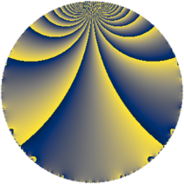# Properties

 Label 91.2.qLevel $91$ Weight $2$ Character orbit 91.q Rep. character $\chi_{91}(36,\cdot)$ Character field $\Q(\zeta_{6})$ Dimension $12$ Newform subspaces $1$ Sturm bound $18$ Trace bound $0$

# Related objects

## Defining parameters

 Level: $$N$$ $$=$$ $$91 = 7 \cdot 13$$ Weight: $$k$$ $$=$$ $$2$$ Character orbit: $$[\chi]$$ $$=$$ 91.q (of order $$6$$ and degree $$2$$) Character conductor: $$\operatorname{cond}(\chi)$$ $$=$$ $$13$$ Character field: $$\Q(\zeta_{6})$$ Newform subspaces: $$1$$ Sturm bound: $$18$$ Trace bound: $$0$$

## Dimensions

The following table gives the dimensions of various subspaces of $$M_{2}(91, [\chi])$$.

Total New Old
Modular forms 24 12 12
Cusp forms 16 12 4
Eisenstein series 8 0 8

## Trace form

 $$12q + 4q^{4} - 18q^{6} - 4q^{9} + O(q^{10})$$ $$12q + 4q^{4} - 18q^{6} - 4q^{9} + 12q^{10} + 6q^{11} - 4q^{12} + 4q^{13} - 8q^{14} + 6q^{15} - 8q^{16} - 4q^{17} - 12q^{20} + 6q^{22} - 12q^{23} + 12q^{24} - 20q^{25} - 42q^{26} + 12q^{27} + 8q^{29} + 8q^{30} + 36q^{32} - 30q^{33} + 6q^{35} - 10q^{36} - 42q^{37} + 4q^{38} - 4q^{39} + 92q^{40} + 30q^{41} + 4q^{42} + 2q^{43} + 12q^{46} - 2q^{48} + 6q^{49} - 18q^{50} + 52q^{51} + 2q^{52} - 44q^{53} + 12q^{54} - 6q^{55} - 12q^{56} - 12q^{58} + 18q^{59} + 14q^{61} - 4q^{62} + 12q^{63} - 52q^{64} + 60q^{65} - 52q^{66} - 24q^{67} - 8q^{68} + 4q^{69} - 24q^{71} + 60q^{72} + 6q^{74} + 46q^{75} - 18q^{76} + 8q^{77} - 10q^{78} - 56q^{79} - 72q^{80} + 2q^{81} + 14q^{82} + 18q^{84} - 48q^{85} - 2q^{87} - 14q^{88} - 12q^{89} + 24q^{90} + 14q^{91} + 24q^{92} - 18q^{93} + 4q^{94} - 22q^{95} + 6q^{97} + O(q^{100})$$

## Decomposition of $$S_{2}^{\mathrm{new}}(91, [\chi])$$ into newform subspaces

Label Dim. $$A$$ Field CM Traces $q$-expansion
$$a_2$$ $$a_3$$ $$a_5$$ $$a_7$$
91.2.q.a $$12$$ $$0.727$$ 12.0.$$\cdots$$.1 None $$0$$ $$0$$ $$0$$ $$0$$ $$q+(-\beta _{1}-\beta _{4}-\beta _{6}+\beta _{10})q^{2}+(-\beta _{2}+\cdots)q^{3}+\cdots$$

## Decomposition of $$S_{2}^{\mathrm{old}}(91, [\chi])$$ into lower level spaces

$$S_{2}^{\mathrm{old}}(91, [\chi]) \cong$$ $$S_{2}^{\mathrm{new}}(13, [\chi])$$$$^{\oplus 2}$$